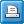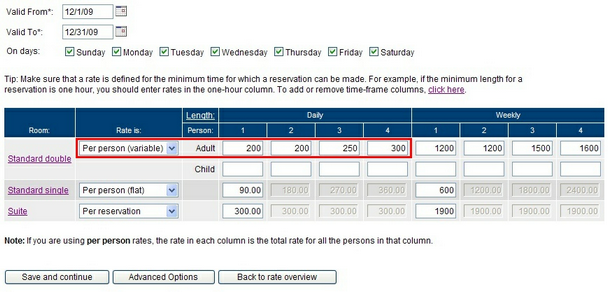﻿ Rates: Per person (variable)
WebReserv User Guide

Rates: Per person (variable)

 Rates: Per person (variable)Rates: Per person (variable)If the rate is per person (variable), the booking system will can use different rates depending on the number of people. To calculate the total rate, the system will multiply the rate with the duration of the reservation.

Number of days x rate (for number of persons) = total rate

Example: If the rate is set to per person (variable) and a reservation is made for 3 persons for 2 days, the total rate will be

2 (days) x 250 (rate for 3 persons) = 500Back to edit rate list